## The Basics of Rotational Motion

At the root of it, rotational motion is just like translational motion. Got it? Good, next chapter.

Well, okay, there are some subtleties. But the key thing to remember is that all that time and energy you put into learning how things slide on tables or get fired out of cannons is still totally valid—with a twist. (Get it? Twist? Because it's rotational? Eh? Fine, never mind.)

Instead of position, rotational motion deals with changes in angle (θ). An object will always rotate—that is, spin or move in a circle—around an axis of rotation. The earth spins around on the axis that runs from the North Pole to the South Pole, creating day and night; figure skaters spin around on the axis from their head to their feet, creating dizzying gold medals; Star Fox spins around on the axis from his spaceship's nose to stern, creating DO A BARREL ROLLs.The axis of rotation, however, can also be outside the rotating object. The sun, for example, is on the axis that the earth rotates around in its orbit.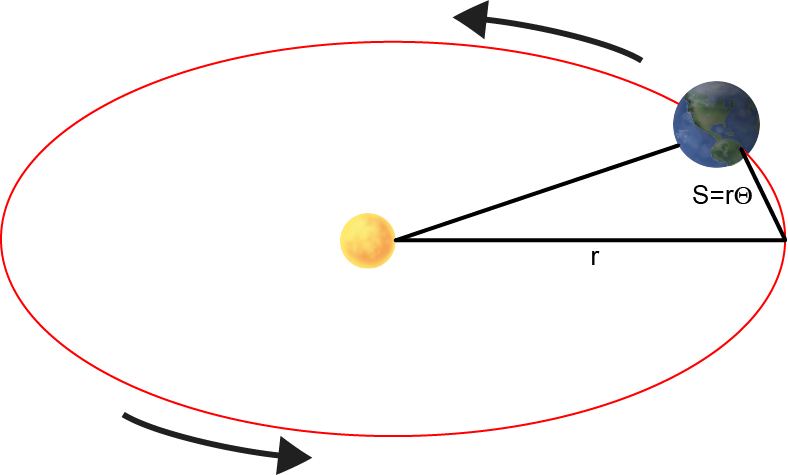An angle is just a way to measure how much exactly an object has spun, or how far around the circle an object has gotten. Of course, we could also measure the distance around the circle in terms of position (say, along the circumference), but angle is a very convenient shorthand, and handy if we're more concerned about the details of the rotation than the translation. Hint: in this chapter, we're more concerned with rotation than translation.

Since angle and position give us the same information, we can convert between the two if we know the radius of the circle. An object that rotates through an angle θ will move along an arc of length:

s =

Here, r is the radius of the circle the object travels along. Both s and r are measured in meters, so angle is technically dimensionless. As a bookkeeping tool, we measure angle in radians. This is similar to the degrees you're probably familiar with—90° is a right angle or a quarter circle, 180° is a change in direction or a half circle, etc.—but on a different scale. Whereas 1° is equal to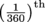of a circle (since a full rotation is 360°), 1 rad is equal to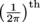of a circle: radians break a circle down into 2π parts, instead of 360. Unfortunately, "2π heelflip" doesn't have quite the same ring as a "360 heelflip", but we'll tackle that problem as soon as a physicist wins an X-Games medal.

Here's the radian equivalent of some common angles:You can translate radians into degrees by multiplying by, but in time you'll learn to love the radian as much as us.* Plus you don't have a choice, since we'll be using it in this chapter, and so Stockholm syndrome is going to help a lot.

### Angular Velocity

So if we measure angle instead of position in rotational motion, we need to know how angle is changing as objects move. Enter angular velocity (ω) and angular acceleration (α). Angular velocity is measured in rad/s, the same way translational velocity is measured in m/s, and represents the change of angle in a given time. A car may be driving at 30 m/s, but its wheels are spinning at 130 rad/s.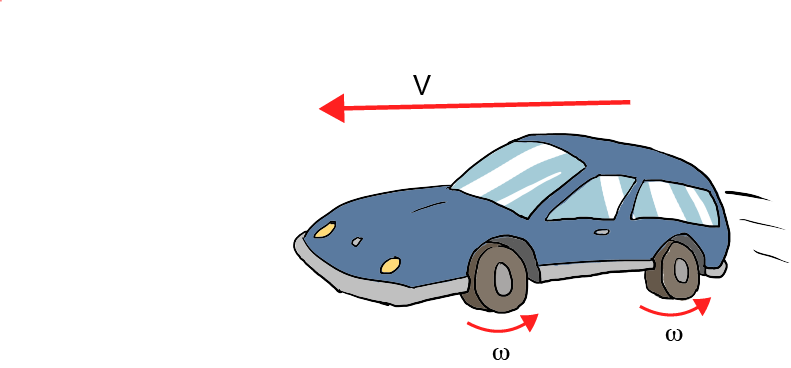Angular acceleration is the rate of change of angular velocity, measured in rad/s2, just as translational acceleration is measured in m/s2. As the car speeds up, the wheels spin faster.

Angular velocity and angular acceleration can be related to their translational counterparts the same way angle is related to arc distance:

vt =
at =

Note that these formulas give answers for rotational motion in radians. The t subscript here is because these velocities and angles are tangential—they're directed along the line tangent to the circle at the point where our object is currently located.That means vt and at are always changing direction if an object is rotating. (Whether they're also changing magnitude depends on α.) It also means that on a rotating object—like a record—the outside is moving faster than the inside, even though they're rotating at the same angular velocity. Whoa.

That point is really the key difference between rotational and translational motion. An object can move at a constant speed, but change its rotational velocity—such as a figure skater performing a lutz. Another way of stating this is the penny-farthing principle: as a penny-farthing bicycle moves, its wheels must rotate at vastly different speeds, because the radii of the two wheels are very different, but their translational speeds are identical (otherwise the wheels would separate from the frame, and your ironically old-fashioned bicycle is less of a hilarious mode of transportation and more of a hideous tool for generating road rash).

We can relate θ, ω, and α to each other the same way we handled x, v, and a in translational motion. Quick recap of some key ideas and formulas from kinematics below.

• An object traveling at constant speed v for t seconds will move x meters:
x
= vt
• An object that moves from an initial velocity of v0 to a final velocity of v in t seconds has an acceleration of a: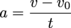• An object that starts at position x0 with initial speed v0 and accelerates at a rate a will end up at position x in t seconds: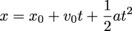• The square of an object's current velocity v is equal to the square of its initial velocity v0 plus two times the product of its current position x and acceleration a:
v2
= v02+2ax

Now, if you add the word "angular" a few times in that list, you end up with a pretty complete formulation of kinematics for rotation. Angular velocity is change in angle, angular acceleration is change of angular velocity, and voila—rotational motion.

• An object traveling at constant angular speed ω for t seconds will turn θ radians:
θ = ωt
• An object that moves from an initial angular velocity of ω0 to a final angular velocity of ω in t seconds has an angular acceleration of α: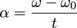• An object that starts at angle θ0 with initial angular speed ω0 and accelerates at a rate α will end up at angle θ in t seconds: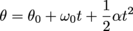• The square of an object's current angular velocity ω is equal to the square of its initial angular velocity ω0 plus two times the product of its current angle θ and angular acceleration α:
ω202+2αθ

### Circular Motion

If an object is constrained to rotate in a circle—say a roller coaster car going around a loop or a ball on a string being twirled above your head—we can say even more about its motion. The force that holds our moving object in a circle, whether its the reaction force of the roller coaster track or the tension of the string, is called centripetal force.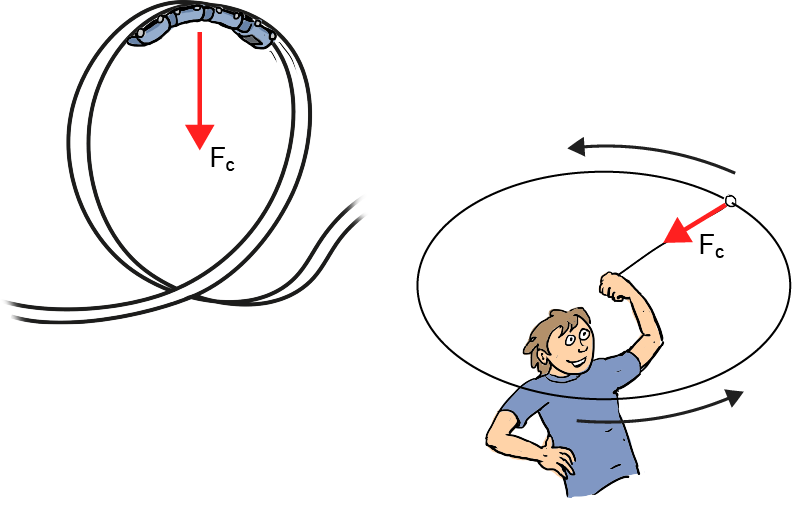Centripetal force always points towards the center of the circle.** Newton's Second Law states centripetal force must have an associated acceleration, called (creatively) centripetal acceleration:

Fc = mac

Centripetal acceleration also points towards the center of a circle. Because of this, it's 90° (ahem,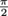rad) away from the rotating object's tangential velocity, and so doesn't affect the object's speed at all—just its direction.The size of ac—and therefore Fc—is directly related to the object's tangential velocity, vt, and the radius of the circle it moves around, r: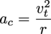The faster an object moves, or the smaller the turn it tries to make, the more force is required to keep it moving in a circle.

This is the theory behind aircraft banked turns—ever been in a plane that takes off in the direction opposite its planned flight path? The plane has to turn around, and does so by banking: tilting its wings into the turn. The lift force on the plane, normally directed vertically, then angles inward.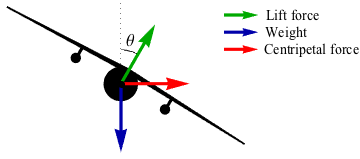The bigger the banking angle, the bigger the horizontal component of the lift force becomes, and the more centripetal force the plane can create, allowing the plane to turn around.

Common Mistakes